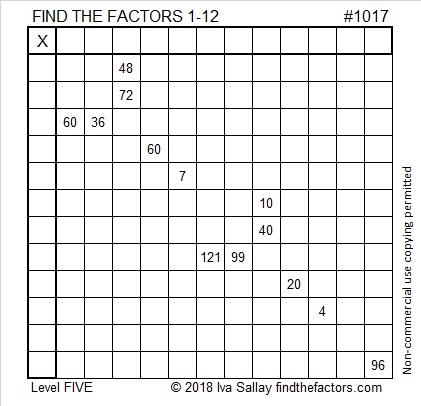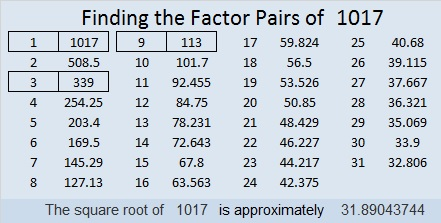# 1017 and Level 5

You might find today’s puzzle to be a little trickier than most level 5 puzzles, but don’t let that deter you from giving it your best effort. For example, it’s true that 6 and 12 are both common factors of 60 and 36, but some of the other clues will eliminate either the 6 or the 12. Can you figure out which one gets eliminated?Print the puzzles or type the solution in this excel file: 12 factors 1012-1018

Let me share some reasons 1017 is an interesting number.

24² + 21² = 1007

1017 is the hypotenuse of a Pythagorean triple:
135-1008-1017 which is 9 times (15-112-113). It can also be calculated
from 24² – 21², 2(24)(21), 24² + 21²

1017 is also palindrome 1771 in BASE 8 because 1(8³) + 7(8²) + 7(8¹) + 1(8⁰) = 1017

• 1017 is a composite number.
• Prime factorization: 1017 = 3 × 3 × 113, which can be written 1017 = 3² × 113
• The exponents in the prime factorization are 2 and 1. Adding one to each and multiplying we get (2 + 1)(1 + 1) = 3 × 2  = 6. Therefore 1017 has exactly 6 factors.
• Factors of 1017: 1, 3, 9, 113, 339, 1017
• Factor pairs: 1017 = 1 × 1017, 3 × 339, or 9 × 113
• Taking the factor pair with the largest square number factor, we get √1017 = (√9)(√113) = 3√113 ≈ 31.890437